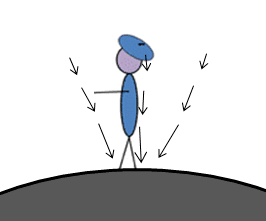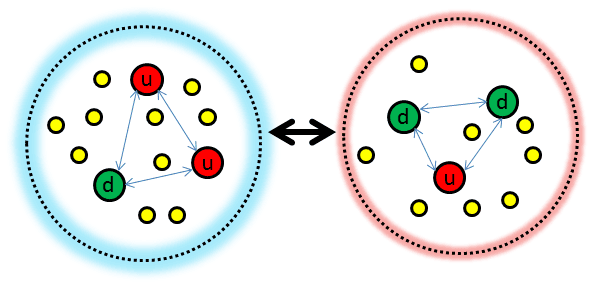# Secondary Forces Explored

[Total: 4    Average: 5/5]

Many are familiar with the “fundamental forces” of nature: gravity, electromagnetism, and the strong and weak nuclear forces. Three of these have a tendency to bind things together in stable ways: as orbits, as atoms, or as nucleons. Once these systems are stable, there are still “residual” effects that the objects can have on one-another through these forces, and this article discusses those.

Tidal Forces

Typically, a gravitationally bound system can be described by an elliptical orbit. Once two things are in orbit around one-another, they no longer feel each others’ gravitational field (depending on how you look at it, this is either a consequence of or the inspiration for the equivalence principle). However, objects that are in freefall around one-another can still influence each other through tidal forces.This person experiences a tidal force because gravity is stronger at their feet than at their beret.

The simplest way to think of a tidal force is that it’s the difference in gravitational field strength between two sides of an object. If some object with length L is a distance R from an object of mass M, we can write down the gravitational field strength on both ends:

$$\Delta g = \frac{GM}{R^2}-\frac{GM}{(R+L)^2}\approx 2\frac{GM}{R^3}L$$

The approximation is from a Taylor expansion in terms of L, truncated by assuming that L is much less than R. We learn two important things about tidal forces from this: they decay with the cube of radius (rather than the square), and they depend on the size of the object experiencing the force. The most well known effect of tidal forces is of course the tides. Here, their inverse-cube dependence explains why the moon and the sun have comparable effects on the Earth: if we compute ##M/r^2## for both bodies, the sun’s effect is 193 times as strong. If we compute  ##M/r^3## the sun’s effect is half that of the moon.

How do tidal forces manifest themselves? They do not cause a center-of-mass acceleration in the way we typically associate with forces, but rather induce a re-shaping of the object in the orbit. It helps to imagine a planet as a ball of fluid, and the tidal field causes the fluid to align along the radial vector of the planet’s orbit. While both the “front” and the “back” of the planet are attracted by the gravitational field, because the “front” feels a stronger attraction, the force on the “back” with respect to the center of mass of the planet is pointed away from the gravitational field. That was hard to word well, so I’ll attach a diagram.Tidal forces in two reference frames. Source.

So, going back to the Earth, the tidal fields of the moon and the sun cause the liquid part of the Earth to reshape to align with the positions of heavenly bodies. Hence, we have ocean tides. There is also a lesser-known effect called Earth Tides, where the planet itself reshapes, resulting in a daily shift in the radius of the Earth by about a centimeter, and a change in the Earth’s gravitational field of about one part in ten million. So if you’re wondering if the sun and the moon’s gravity effects you, it does, but indirectly.

When tidal fields reshape rotating planets or moons, this can effect how those objects rotate. When an object is elongated, it is in a preferred tidal configuration, and as it rotates out of that configuration, there is a residual torque resisting its rotation, which over long periods of time slows down the rotation period until it matches the orbital period, such that the object can always have its preferred* configuration in the tidal field. This is why the same side of the moon always points at the Earth, and why over long periods of time the Earth’s rotation is slowing down, until its same side will always be pointing at the moon**.

Dipole and van der Waals interactions.

At the classical level, the gravitational and electrostatic forces are pretty similar. Besides the very large difference in strength, magnetic effects are quite relevant while gravitomagnetism is not, and there is only one kind of gravitational charge (mass) but there are positive and negative electric charges, and this makes the biggest difference. As you delve deeper into the understanding of each phenomenon, quantum electrodynamics does not bear much resemblance to general relativity, despite Newton’s and Coulomb’s laws being almost the same.

When a positive nucleus and negative electrons get together, you have an atom, and this is the bound electrostatic state that I am analogizing to a gravitational orbit. Between a nucleus and its electrons there are effects analogous to tidal fields, including spin-orbit coupling that leads to fine and hyperfine atomic structure (slightly different energy levels depending on the configurations of various angular momenta), which is typically taught, at least in earlier quantum classes, in terms of a magnetic force that the electron feels due to the nucleus “orbiting” around it.

When a positive and negative charge are near one another, the total electric field far from the pair (dipole) is zero, but closer to the dipole, the electric field might be stronger or weaker and positive or negative depending on whether you’re closer to the positive or negative end of it. With the same reasoning used to derive the inverse-cube tidal force, you can show that the dipole electric field decays as the inverse cube of distance, but there is additional angular dependence which you can derive if you want. If you have two dipoles near each other, they can rotate so that they are favourably aligned, and then attract each other.A diagram of dipolar attractions between molecules. Source.

These dipole interactions are responsible for attraction between atoms and molecules, in liquids and non-covalent solids. Because dipole electric fields decay as the inverse cube, and the attraction due to a dipole field decays with an additional cube, the attractive interaction energy decays as the sixth power of distance, which is pretty weak. There are various forms of intermolecular dipole attraction, but they are generally referred to as van der Waals forces, and consist of, from strongest to weakest, forces between permanent dipoles (Keesom forces), forces between a permanent dipole and a non-polar molecule (Debye forces), and forces between non polar atoms or molecules (London forces). Although this may not be established formally, the induced dipoles associated with the latter can be imagined as analogous to the torque induced in a rotating moon by a tidal field: it didn’t have the asymmetry required for spin-orbit coupling until it was induced by the gravitational field gradient.A preferred crystalline configuration of many van der Waalsing polar atoms. Source.

When non-bonded atoms get too close to one-another, the electrons resist occupying the same state, and this gives them a short-range repulsive force. The inverse-sixth attraction decays pretty strongly, but the repulsion is usually described by an inverse-twelfth or exponentially decaying energy term, and together these are described by the Lennard-Jones potential***, which is useful for modelling condensed matter systems.

How strong are dipolar intermolecular attractions? One way to gauge this is by looking at the tabulation “enthalpy of vaporization” which tells you how much energy is required to separate a mole of aggregated atoms or molecules, and then divide by Avogadro’s number to find the binding energy per molecule. For (arbitrarily chosen) nitrogen, this is about 5 kilojoules per mole, or about 50 milli-electron volts per molecule. For water, it is about eight times as strong, but there is also hydrogen bonding to contend with. For a covalently bonded aggregate like carbon, the heat of vaporization corresponds to roughly 3 eV, a typical outer-electro/atom binding energy. I would say this is a very “zeroth order” way to look at chemical physics, but it gives you a sense of how strong these attractions are.

The Nuclear Force

One might read that the strong nuclear force is responsible for holding protons and neutrons together in nuclei. This is partially true, but not completely accurate. The strong nuclear force is responsible for holding quarks together to form proton and neutrons. But the protons and neutrons themselves are held together by the residual strong force, which is sometimes just called the nuclear force. It is also called the Yukawa interaction, after the Japanese physicist who formulated it. Personally, I like the analogy that the Yukawa interaction is the van der Waals version of the strong force, where larger-scale attractions arise due to residual effects after bound states have been formed. Particle interactions are often described in terms of exchange of an intermediary particle, and in the case of this interaction it is the pion, a quark-antiquark pair, that is transferred between protons and neutrons. Wikipedia has a cool gif of this which confuses me a little. Because the intermediary particle has mass, the potential has an exponentially decaying coefficient on top of a Coulomb 1/r, which makes it very short range.A rough schematic of a proton and neutron, which are held together by the strong attraction between quarks (blue arrows), are bound to one another through the residual nuclear force. I have tried to avoid the “protons are triangles” misconception.

The weak force sucks. Neutrinos are cool though.

Conclusion.

I have discussed secondary phenomena that lead to residual interactions after a “primary” force has created a bound state. The three examples are not all described by the same physics, but conceptually I feel that they have a lot in common.

*Preferred meaning lowest-energy.

**Unless the sun dies first.

***John Lennard-Jones should not be confused with Philipp Lenard, who was one of Hitler’s chief physicists.

6 replies
1.Useful nucleus says:

Truly insightful, thanks for sharing !

2.create14all says:

This is an interesting notion.When tidal fields reshape rotating planets or moons, this can effect how those objects rotate. When an object is elongated, it is in a preferred tidal configuration, and as it rotates out of that configuration, there is a residual torque resisting its rotation, which over long periods of time slows down the rotation period until it matches the orbital period, such that the object can always have its preferred* configuration in the tidal field. This is why the same side of the moon always points at the Earth, and why over long periods of time the Earth’s rotation is slowing down, until its same side will always be pointing at the moon**.Reference https://www.physicsforums.com/insights/secondary-forces/Do you have any calculations or further explanations?

3.Drakkith says:

Nice article!

The weak force sucks. Neutrinos are cool though.

:roll:

4.Greg Bernhardt says:

Nice article!
:roll:

@Orodruin will be satisfied :biggrin:

5.klotza says:

Truly insightful, thanks for sharing !

6.create14all says:

This is an interesting notion.

When tidal fields reshape rotating planets or moons, this can effect how those objects rotate. When an object is elongated, it is in a preferred tidal configuration, and as it rotates out of that configuration, there is a residual torque resisting its rotation, which over long periods of time slows down the rotation period until it matches the orbital period, such that the object can always have its preferred* configuration in the tidal field. This is why the same side of the moon always points at the Earth, and why over long periods of time the Earth’s rotation is slowing down, until its same side will always be pointing at the moon**.

Do you have any calculations or further explanations?

7.klotza says:

You’re looking for more detailed calculations about tidal locking?

Maybe here: [URL]http://www.astro.sunysb.edu/lattimer/AST301/lecture_tide.pdf[/URL]